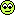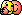## [.uhm] FM Synthesis in Hive

Official support for: u-he.comUrs
u-he
23864 posts since 8 Aug, 2002 from Berlin
Just like the Karplus-Strong thread, this one is for FM Synthesis, or, to be precise, "Delta X", the phase modulation synthesis which gave the Yamaha DX series its name.

Again, you need the Hive Tech Preview thread and ist downloads a pre-requisites:

http://www.kvraudio.com/forum/viewtopic ... 1&t=511390

Bypassing further ado, let's dive right in:

Chapter 1: FM Fundamentals

The fundamental idea of FM is to take a sine wave oscillator (modulator) to modulate the *phase* of another sine wave oscillator (carrier). What you hear is the carrier, which has a richer spectrum than two sine waves would suggest. The spectrum (or resulting waveform) depends on the frequency relation of those two oscillators as well as on the depth/amplitude of the phase modulation. Sonic movement similar to filter sweeps in classic subtractive synthesis is achieved by scaling the volume of the modulator signal with envelopes. Disharmonic sounds (bells etc.) can be achieved by altering pitch, or simple by having a non-integer frequency relation of modulator and carrier.

While Wavetables can of course only ever have harmonic content, there's still a whole lot of FM goodness available.

Here's a simple sine wave oscillator:

Code: Select all

``Wave "sin(2*pi*phase)"``
Remember that sin/cos etc. expect values in Radians, so we have to scale our phase by 2*pi. This is our fundamental building block.

Now, let's create another sine, but of any harmonic of choice:

Code: Select all

``Wave "sin(2*pi*phase * 16)"``
Yep, that's the 16th harmonic (fundamental being the 1st).

Now, let's see how to move phase, since that's what we want to modulate:

Code: Select all

``Wave "sin(2*pi*phase + table*2*pi)"``
When you run this one, the sine wave moves from right to left. Each frame has a different phase.

Lesson: *Multiply* 2*pi*phase by n to change harmonic. *Add* 2*pi*n to change phase.

The general FM operator

So, in general our FM operators consist of the following formula:

sin( 2*pi*phase*harmonic + 2*pi*delta*depth )

optionally shortened to

sin( 2*pi* (phase*harmonic + delta*depth) )

Where harmonic is a whole number (1,2,3,4...16 or so) and delta is the output of another operator, optionally scaled by a depth parameter (Typically between 0 and, maybe, 5 or so, but that's up to anyone).

Note: I wrote harmonic, delta and depth in italics because we need to replace them with variables or functions available in the scripting language.

Examples!

Here's the simple most FM wavetable .uhm script:

Code: Select all

``Wave "sin(2*pi*phase + sin(2*pi*phase*4))"``
This one creates a dull static squar-ish waveform. Not much movement. Also, while it's nice to have it all in one line, let's start making it tidy.

Code: Select all

``````Wave "sin(2*pi*phase*4)"
Wave "sin(2*pi*phase + x)"``````
^^ This is the same, but each operator gets its own line. The "x" always contains the value written to the wavetable in previous lines, so it's a good way to separate things. It also coincides with the x in DX (but that really is just coincidence).

Now, let's modulate the depth.

Code: Select all

``````Wave "sin(2*pi*phase*4)"
Wave "sin(2*pi*phase + x*(1-table))"``````
Yep, now we're getting somewhere. It sweeps.

It is still a bit boring though. If you look at patch sheets from FM synths, they usually have Time/Level envelopes, shaping a nice short initial Decay to some low value, followed by a slow long decay towards zero. FM is renowned for its percussive sounds.

Hence:

Envelopes!

Of course you can do envelopes any way you like. You can create your wavetables bit by bit using the start/end frame positions. But that's tedious. Hence there is a 8-segment Time/Level envelope built into the engine. It nicely lends itself to FM Synthesis.

Code: Select all

``````Info "Simple FM patch"
NumFrames=101

/*
Introducing: The Envelope Feature!

No. | Time | Level
0   |  --- |  1.0
1   |  0.2 |  0.3
2   |  0.8 |  0.0
...
8   |      |
*/

Envelope L0=1 T1=0.2 L1=0.3 T2=0.8 L2=0.0

Wave "sin( 2*pi*phase * 5 )" // Modulator on 5th harmonic

Wave "sin( 2*pi*(phase + x * env(table)) )"``````
Now, let's check this out:

Code: Select all

``Envelope L0=1 T1=0.2 L1=0.3 T2=0.8 L2=0.0``
We can create a Time/Level envelope, starting at Level 0, followed by pairs of Time and Level values 1-8

Before you ask: This envelope only exists in the script. It does not magically appear in Hive somewhere.

The way you use it, is in the last line. The envelope is accessed by the env(...) function in the formula parser:

Code: Select all

``Wave "sin( 2*pi*(phase + x * env(table)) )"``
You can use *any* variable, formula whatsoever as time base for the envelope. If you use "table", the envelope will neatly evolve from first frame to last, as long as the Time values are between 0 and 1. You can also use env(frame) for envelopes where the times are set up to have things happen at specific frames. In general I prefer "table" though, as I can change the NumFrames afterwards without affecting the envelope.

(and yes, you can use "phase" as time base as well, in case of which the envelope evolves over the course of the cycle... see "Tut03 Classic Waves Part 3.uhm" for creating waveforms using the envelope.)

Now. Let that sink in. I'll think of something for the next chapters. More operators? Layering? Feedback modulation?EvilDragon
KVRAF
18595 posts since 7 Jan, 2009 from Croatia
Urs wrote:More operators? Layering? Feedback modulation?
RCM from SY77/99.Howard
KVRAF
3797 posts since 23 May, 2004 from Bad Vilbel, Germany
EvilDragon wrote:RCM from SY77/99.I only got trash when using AWM as modulator. No wonder people didn't take to it.bmrzycki
KVRAF
4112 posts since 11 Aug, 2006 from Austin, TX
Urs, what is the time unit for the envelope Tn= feature?
Feel free to call me Brian.Urs
u-he
23864 posts since 8 Aug, 2002 from Berlin
bmrzycki wrote:Urs, what is the time unit for the envelope Tn= feature?
Here's the thing you need an a-ha moment for: It's up to you! It's whatever t you put into env(t)

If you want to use the envelope to draw a triangle wave, you might want to make it go from 0 to 2047, because that's what index travels through:

Code: Select all

``````Envelope L0=0 T1=512 L1=1 T2=1024 L2=-1 T3=512 L4=0
Wave "env(index)"``````
or you go by phase, which is in the tutorial script:

Code: Select all

``````Envelope L0=0 T1=0.25 L1=1 T2=0.5 L2=-1 T3=0.25 L4=0
Wave "env(phase)"``````
There are numerous applications for it. Whenever phase, index whatever is too "linear" for the task at hand, the envelope feature might just do the trick!Urs
u-he
23864 posts since 8 Aug, 2002 from Berlin
Also, you can almost directly translate DX-7 patch sheets to .uhm envelopes (less key scaling and stuff, but still...)EvilDragon
KVRAF
18595 posts since 7 Jan, 2009 from Croatia
Howard wrote:
EvilDragon wrote:RCM from SY77/99.I only got trash when using AWM as modulator. No wonder people didn't take to it.
Yeah using raw samples would be trash, but if you filter them before hitting an operator...drzhnn
KVRist
476 posts since 1 Jan, 2013 from Saint-Petersburg, Russia
I really like the brightness and clearness of the uhm scripts. Very crisp, firm and stable. And there's no spectral leakage or any degradation and artifacts when playing very low and very high notes. Really impressive stuff.

Code: Select all

``````Info "FM thing that uses two envelopes"
NumFrames = 10

Seed "71915651"
Envelope L0=0.3 T1=0.2  L1=1.0 T2=0.8  L2=0.03 // simple AD envelope
Spectrum lowest=1 highest=16 "env(randf + table) * phase * pi" // it started as env(table) but then I threw in some other stuff just for fun and it worked
Wave start=6 "bandpass(x, 0.3, 0.9)" // I didn't like the transition in frames 6-10, so I altered them with bandpass filter

Envelope L0=0.3 T1=0.5  L1=0.0 T2=0.0  L2=1.0 T3=0.1  L3=0.0 T4=0.0  L4=0.35 T5=0.3  L5=0.05 T6=0.0  L6=0.1 T7=0.1  L7=0.3 // I'm feeling so smart right now
Wave start=2 "sin(2*pi * (phase + (x * env(table))))" // FMing starts from frame 2 to add some extra movement

Normalize base=each
``````hollo
KVRian
992 posts since 28 Dec, 2004
Urs wrote:Also, you can almost directly translate DX-7 patch sheets to .uhm envelopes (less key scaling and stuff, but still...)
Your Dx Piano.uhm is just Brilliant Urs!I was playing a lot with it today and it is working and reacting to key and velocity like a charm. Like the real thing.

Now I beginning to understand that you'v put more operators and different envelopes into the script.

OMG! What will coming to us from this Script.
I'v already put away all my work and can't stop playing with the wavetables.
Wavetables get a new meaning now, how can it be so fresh and clean.

Wavetable HD 4kpdxindy
KVRAF
16025 posts since 3 Feb, 2005 from in the wilds
drzhnn wrote:I really like the brightness and clearness of the uhm scripts. Very crisp, firm and stable. And there's no spectral leakage or any degradation and artifacts when playing very low and very high notes. Really impressive stuff.
Yeah! The low and high notes sound lovely... so clean bright and clear... yet not harsh or cold. Very impressive...Urs
u-he
23864 posts since 8 Aug, 2002 from Berlin
hehehe... that's why I was dying to publish this asap... glad you like itEvilDragon
KVRAF
18595 posts since 7 Jan, 2009 from Croatia
DX Piano wavetable + 8 voice unison and some detuning = instant TX816!Urs
u-he
23864 posts since 8 Aug, 2002 from Berlin
EvilDragon wrote:DX Piano wavetable + 8 voice unison and some detuning = instant TX816!Delta Sign
KVRian
655 posts since 22 Jun, 2018
A simple 5OP FM script I made as a second layer for the additive preset I posted in the other thread :

Code: Select all

``````NumFrames=256

Wave "sin(2*pi*phase*7)
Envelope curve=quadric L0=0.03 T1=0.1 L1=0
Wave "sin(2*pi*(phase+x*env(table))*5)"
Envelope curve=quadric L0=0.2 T1=0.2 L1=0
Wave "sin(2*pi*(phase+x*env(table))*2)"
Envelope curve=quadric L0=0.1 T1=1 L1=0.2
Wave "x*env(table)"
Envelope curve=quadric L0=0 T1=0.5 L1=0.05 T2=0.5 L2=0Hehehe, you're moving faster than I can keep up with